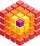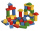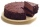# Cube + prism - math problems

#### Number of problems found: 23

• Mike choseMike chose 4 identical cubes, 3 identical prisms and 2 identical cylinders from the kit. The edge of the cube is 3 cm long. The prism has two dimensions the same as the cube, its third dimension is 2 times longer. The diameter of the base of the cylinder
• Regular square prismThe volume of a regular square prism is 192 cm3. The size of its base edge and the body height is 1: 3. Calculate the surface of the prism.
• KarolínaKarolína chose 5 bodies from the kit - white, blue and gray cubes, a blue cylinder and a white triangular prism. How many different roof towers can be built one by one if all the blue bodies (cube and cylinder) are not placed on top of each other?
• Cube-shaped containerThe cube-shaped container has a height of 52 cm and a square base. The container was filled to the brim with water, then we immersed a metal cube in it, which caused 2.7 l of water to flow out of the container. After removing the cube from the water, the
• Wooden blockA cuboid-shaped wooden block has 6 cm length, 4 cm breadth, and 1 cm height. Two faces measuring 4 cm x 1 cm are colored in black. Two faces measuring 6 cm x 1 cm are colored in red. Two faces measuring 6 cm x 4 cm are colored in green. The block is divid
• Cutting the prismA prism with a square base with a content of 1 cm2 and a height of 3 cm was cut from a cube with an edge length of 3 cm. What is the surface of the body formed from the cube after cutting the prism?
• Identical cubesFrom the smallest number of identical cubes whose edge length is expressed by a natural number, can we build a block with dimensions 12dm x 16dm x 20dm?
• Alien shipThe alien ship has the shape of a sphere with a radius of r = 3000m, and its crew needs the ship to carry the collected research material in a cuboid box with a square base. Determine the length of the base and (and height h) so that the box has the large
• Wooden containerThe locksmith should cover the cube-shaped wooden container with a metal sheet inside. The outer edge of the container is 54cm. The wall thickness is 25 mm. The container has no lid. Calculate. How many sheets will be needed to cover it?
• Cube cutIn the ABCDA'B'C'D'cube, it is guided by the edge of the CC' a plane witch dividing the cube into two perpendicular four-sided and triangular prisms, whose volumes are 3:2. Determine in which ratio the edge AB is divided by this plane.
• DortThe cube-shaped cube box has dimensions of 30 cm × 30 cm × 12 cm. How many CZK (Czech crowns) would cost be the biggest cake that would fit into the box when a 10 cm³ cake costs CZK 0.5? The cake has the same shape as the box.
• Cubes into cuboidHow many 12 centimeter cubes fit into the block (cuboid) with 6dm, 8.4dm, and 4.8?
• Surface of cubesPeter molded a cuboid 2 cm, 4cm, 9cm of plasticine. Then the plasticine split into two parts in a ratio 1:8. From each piece made a cube. In what ratio are the surfaces of these cubes?
• Water blockA block with a 50 cm2 base is filled with water 5 cm under the edge. How many can sugar cubes with 2 cm edge be thrown into a container that overflow water?
• Cuboid edges in ratioCuboid edges lengths are in ratio 2:4:6. Calculate their lengths if you know that the cuboid volume is 24576 cm3.
• Scale factorA prism with a volume of 1458 mm3 is scaled down to a volume of 16mm3. What is the scale factor in fraction form?
• Rectangle poolFind dimensions of an open pool with a square bottom with a capacity of 32 m3 to have painted/bricked walls with the least amount of material.
• Chocolate rollThe cube of 5 cm chocolate roll weighs 30 g. How many calories will contain the same chocolate roller of a prism shape with a length of 0.5 m whose cross-section is an isosceles trapezoid with bases 25 and 13 cm and legs 10 cm? You know that 100 g of this
• Prism basesVolume perpendicular quadrilateral prism is 360 cm3. The edges of the base and height of the prism are in the ratio 5:4:2. Determine the area of the base and walls of the prism.
• Tetrahedral prismThe height of a regular tetrahedral prism is three times greater than the length of the base edge. Calculate the length of the base edge, if you know that the prism volume is 2187 cm3.

Do you have an exciting math question or word problem that you can't solve? Ask a question or post a math problem, and we can try to solve it.

We will send a solution to your e-mail address. Solved examples are also published here. Please enter the e-mail correctly and check whether you don't have a full mailbox.

Cube Problems. Prism Problems.